# Linear Equations Standard Form Graphs to equations Equations

• Slides: 17Linear Equations Standard Form Graphs to equations Equations to Graphs Finding values Problem solving 100 100 100 500 500 500 1000 1000 Bonus Question: 5000 pts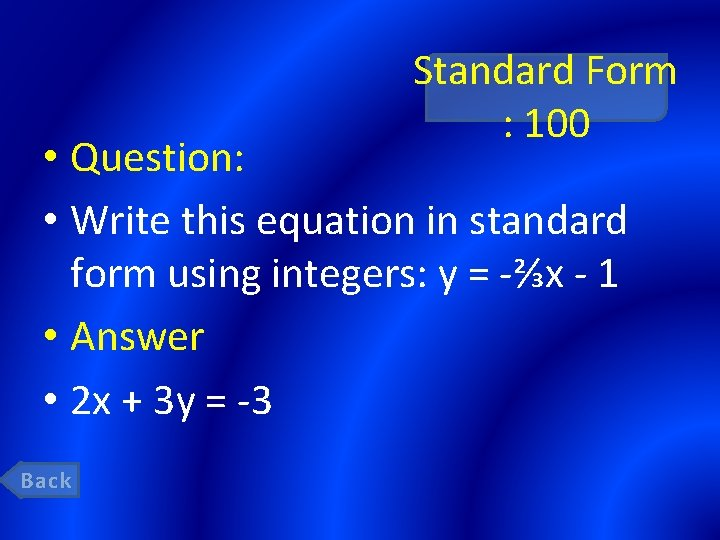Standard Form : 100 • Question: • Write this equation in standard form using integers: y = -⅔x - 1 • Answer • 2 x + 3 y = -3 Back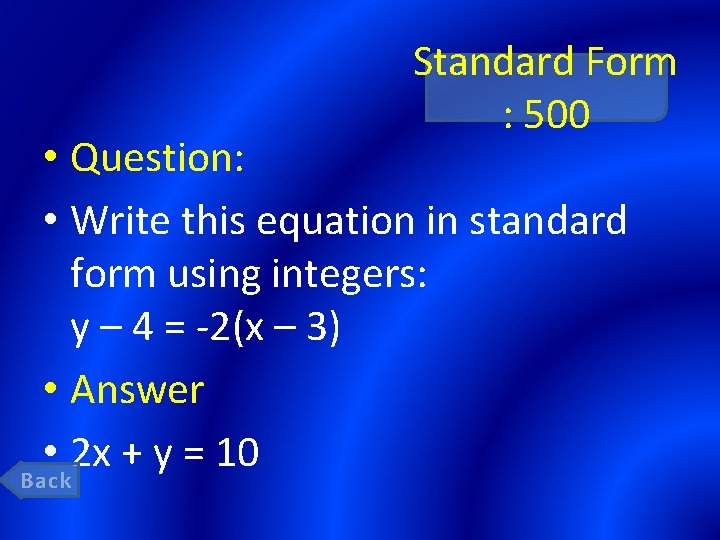Standard Form : 500 • Question: • Write this equation in standard form using integers: y – 4 = -2(x – 3) • Answer • 2 x + y = 10 BackStandard Form : 1000 • Question: • CHALLENGE QUESTION: Write an equation in standard form of a line that contains the point (-4, -7) and has the same slope as the graph of y + 3 = 5(x + 4) • Answer • First, put in point slope: y + 4 = 5(x + 7) Then, put in standard form: – 5 x + y = 31 Back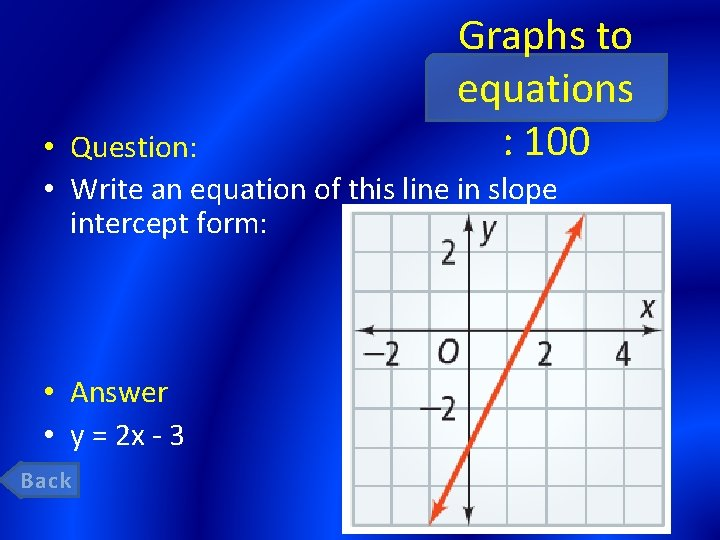Graphs to equations : 100 • Question: • Write an equation of this line in slope intercept form: • Answer • y = 2 x - 3 BackGraphs to equations : 500 • Question: • Write an equation of this line in point-slope form. Label which point you use. • Answer • y – 2 = (5/2) (x – 1) [answers will vary] BackGraphs to equations 1000 : • Question: • Write an equation for this line in slope-intercept form, point-slope form using (3, 4), AND standard form using integers. Back • Answer • Slope-intercept: y = -⅔x + 6 Point-slope: y – 4 = -⅔ (x – 3) Standard: 2 x + 3 y = 18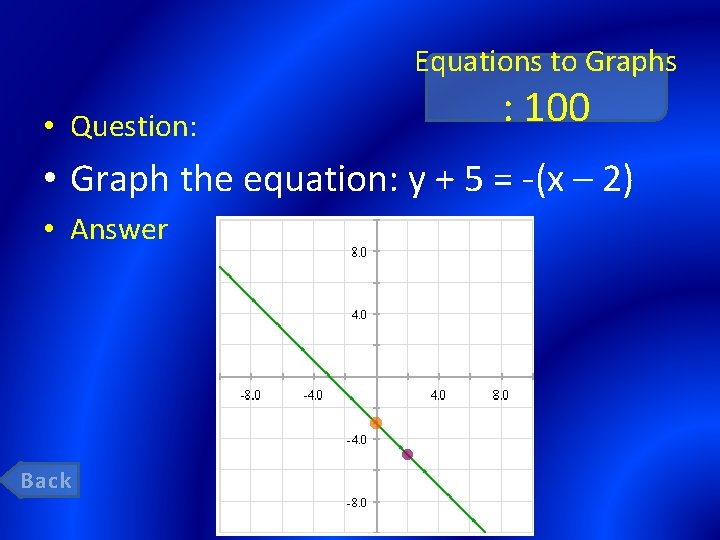Equations to Graphs • Question: : 100 • Graph the equation: y + 5 = -(x – 2) • Answer Back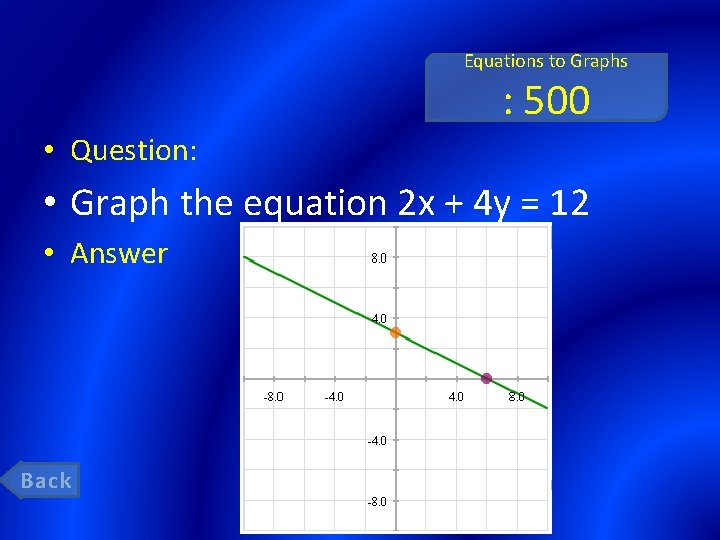Equations to Graphs : 500 • Question: • Graph the equation 2 x + 4 y = 12 • Answer Back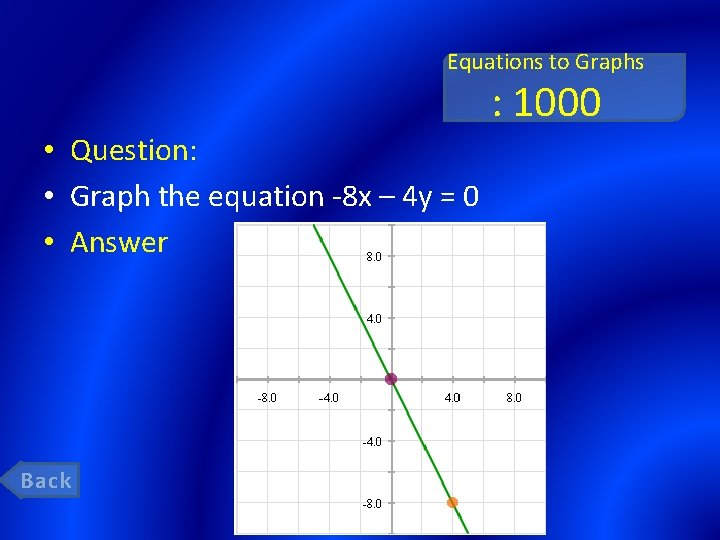Equations to Graphs : 1000 • Question: • Graph the equation -8 x – 4 y = 0 • Answer Back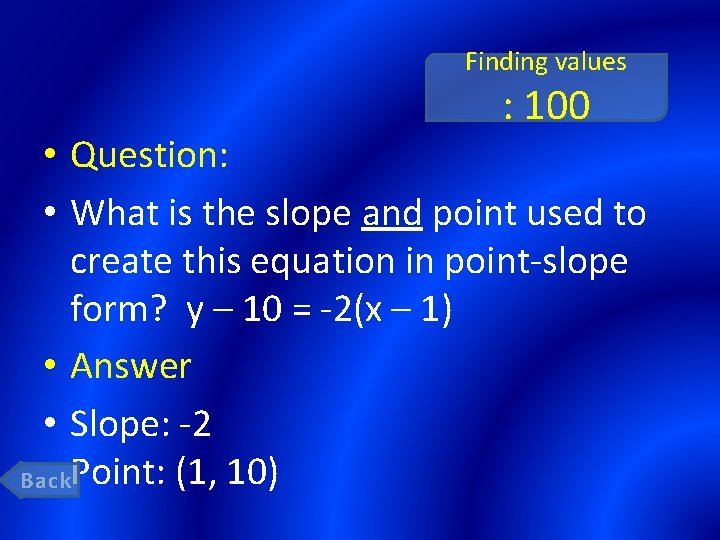Finding values : 100 • Question: • What is the slope and point used to create this equation in point-slope form? y – 10 = -2(x – 1) • Answer • Slope: -2 Back. Point: (1, 10)Finding values : 500 • Question: • What are the x- and y- intercepts of this line: 3 x – 5 y = -75 • Answer • x-intercept: -25 y-intercept: 15 BackFinding values : 1000 • Question: • What is the x-intercept, y-intercept, and slope of this line: 2 x + 4 y = 8 • Answer • x-intercept: 4; y-intercept: 2 slope: -½ Back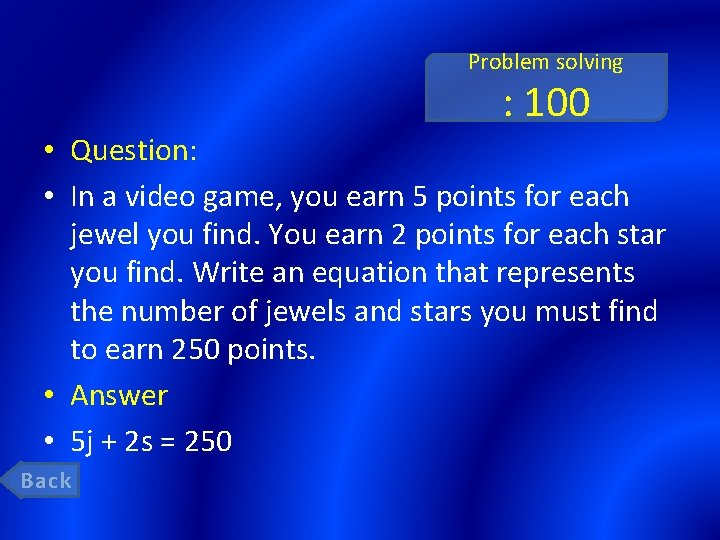Problem solving : 100 • Question: • In a video game, you earn 5 points for each jewel you find. You earn 2 points for each star you find. Write an equation that represents the number of jewels and stars you must find to earn 250 points. • Answer • 5 j + 2 s = 250 Back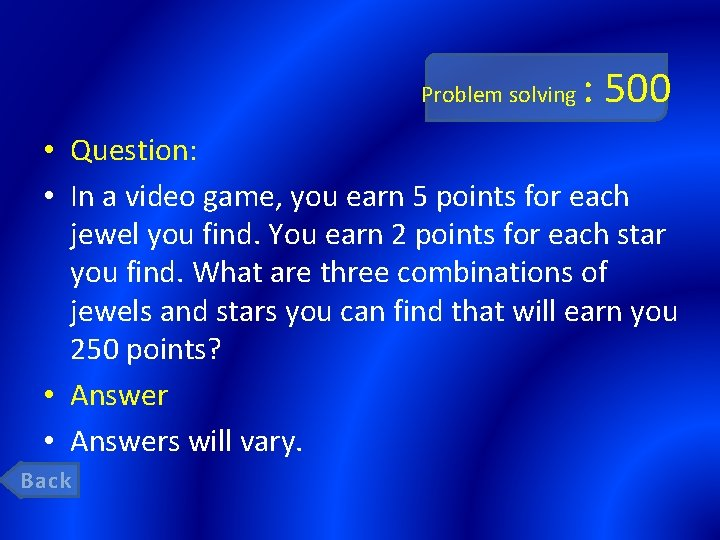Problem solving : 500 • Question: • In a video game, you earn 5 points for each jewel you find. You earn 2 points for each star you find. What are three combinations of jewels and stars you can find that will earn you 250 points? • Answers will vary. Back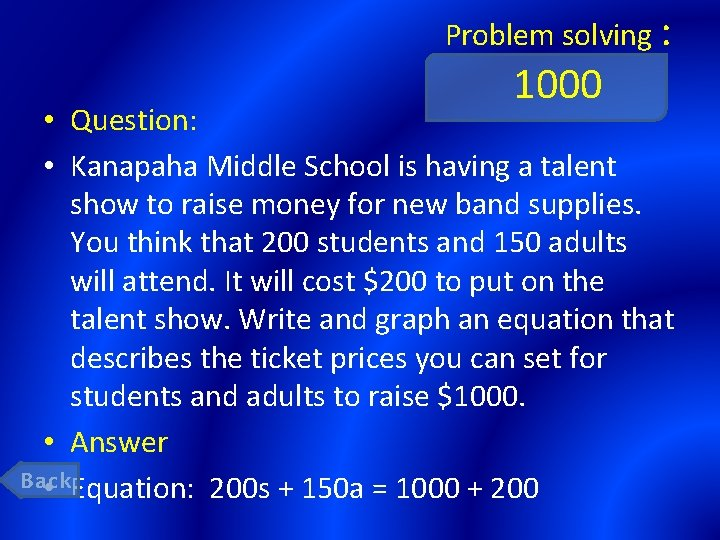Problem solving : 1000 • Question: • Kanapaha Middle School is having a talent show to raise money for new band supplies. You think that 200 students and 150 adults will attend. It will cost \$200 to put on the talent show. Write and graph an equation that describes the ticket prices you can set for students and adults to raise \$1000. • Answer Back • Equation: 200 s + 150 a = 1000 + 200